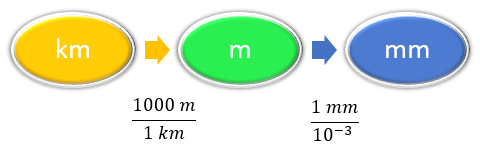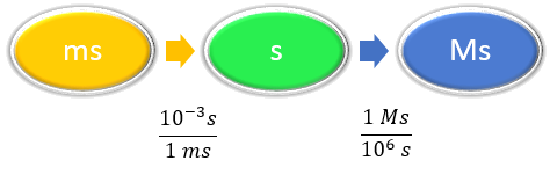# 2.7: Solving Multistep Conversion Problems

$$\newcommand{\vecs}{\overset { \rightharpoonup} {\mathbf{#1}} }$$ $$\newcommand{\vecd}{\overset{-\!-\!\rightharpoonup}{\vphantom{a}\smash {#1}}}$$$$\newcommand{\id}{\mathrm{id}}$$ $$\newcommand{\Span}{\mathrm{span}}$$ $$\newcommand{\kernel}{\mathrm{null}\,}$$ $$\newcommand{\range}{\mathrm{range}\,}$$ $$\newcommand{\RealPart}{\mathrm{Re}}$$ $$\newcommand{\ImaginaryPart}{\mathrm{Im}}$$ $$\newcommand{\Argument}{\mathrm{Arg}}$$ $$\newcommand{\norm}{\| #1 \|}$$ $$\newcommand{\inner}{\langle #1, #2 \rangle}$$ $$\newcommand{\Span}{\mathrm{span}}$$ $$\newcommand{\id}{\mathrm{id}}$$ $$\newcommand{\Span}{\mathrm{span}}$$ $$\newcommand{\kernel}{\mathrm{null}\,}$$ $$\newcommand{\range}{\mathrm{range}\,}$$ $$\newcommand{\RealPart}{\mathrm{Re}}$$ $$\newcommand{\ImaginaryPart}{\mathrm{Im}}$$ $$\newcommand{\Argument}{\mathrm{Arg}}$$ $$\newcommand{\norm}{\| #1 \|}$$ $$\newcommand{\inner}{\langle #1, #2 \rangle}$$ $$\newcommand{\Span}{\mathrm{span}}$$$$\newcommand{\AA}{\unicode[.8,0]{x212B}}$$

## Multiple Conversions

Sometimes you will have to perform more than one conversion to obtain the desired unit. For example, suppose you want to convert 54.7 km into millimeters. We will set up a series of conversion factors so that each conversion factor produces the next unit in the sequence. We first convert the given amount in km to the base unit which is meters. We know that 1,000 m =1 km.

Then we convert meters to mm, remembering that $$1\; \rm{mm}$$ = $$10^{-3}\; \rm{m}$$.

Concept MapCalculation

\begin{align*} 54.7 \; \cancel{\rm{km}} \times \dfrac{1,000 \; \cancel{\rm{m}}}{1\; \cancel{\rm{km}}} \times \dfrac{1\; \cancel{\rm{mm}}}{\cancel{10^{-3} \rm{m}}} & = 54,700,000 \; \rm{mm} \\ &= 5.47 \times 10^7\; \rm{mm} \end{align*}

In each step, the previous unit is canceled and the next unit in the sequence is produced, each successive unit canceling out until only the unit needed in the answer is left.

Example $$\PageIndex{1}$$: Unit Conversion

Convert 58.2 ms to megaseconds in one multistep calculation.

SOLUTION

Steps for Problem Solving

Unit Conversion

Identify the "given"information and what the problem is asking you to "find."

Given: 58.2 ms

Find: Ms

List other known quantities

$$1 ms = 10^{-3} s$$

$$1 Ms = 10^6s$$

Prepare a concept mapCalculate

\begin{align} 58.2 \; \cancel{\rm{ms}} \times \dfrac{10^{-3} \cancel{\rm{s}}}{1\; \cancel{\rm{ms}}} \times \dfrac{1\; \rm{Ms}}{1,000,000\; \cancel{ \rm{s}}} & =0.0000000582\; \rm{Ms} \nonumber\\ &= 5.82 \times 10^{-8}\; \rm{Ms}\nonumber \end{align}\nonumber

Neither conversion factor affects the number of significant figures in the final answer.

Example $$\PageIndex{2}$$: Unit Conversion

How many seconds are in a day?

Solution

Steps for Problem Solving

Unit Conversion

Identify the "given"information and what the problem is asking you to "find."

Given: 1 day

Find: s

List other known quantities

1 day = 24 hours

1 hour = 60 minutes

1 minute = 60 seconds

Prepare a concept mapCalculate

$1 \: \text{d} \times \frac{24 \: \text{hr}}{1 \: \text{d}}\times \frac{60 \: \text{min}}{1 \: \text{hr}} \times \frac{60 \: \text{s}}{1 \: \text{min}} = 86,400 \: \text{s} \nonumber$

Exercise $$\PageIndex{1}$$

Perform each conversion in one multistep calculation.

1. 43.007 ng to kg
2. 1005 in to ft
3. 12 mi to km
$$4.3007 x 10^{-14} kg$$
83.75 ft
19 km

Career Focus: Pharmacist

A pharmacist dispenses drugs that have been prescribed by a doctor. Although that may sound straightforward, pharmacists in the United States must hold a doctorate in pharmacy and be licensed by the state in which they work. Most pharmacy programs require four years of education in a specialty pharmacy school. Pharmacists must know a lot of chemistry and biology so they can understand the effects that drugs (which are chemicals, after all) have on the body. Pharmacists can advise physicians on the selection, dosage, interactions, and side effects of drugs. They can also advise patients on the proper use of their medications, including when and how to take specific drugs properly. Pharmacists can be found in drugstores, hospitals, and other medical facilities. Curiously, an outdated name for pharmacist is chemist, which was used when pharmacists formerly did a lot of drug preparation, or compounding. In modern times, pharmacists rarely compound their own drugs, but their knowledge of the sciences, including chemistry, helps them provide valuable services in support of everyone’s health.A woman consulting with a pharmacist. This image was released by the National Cancer Institute, an agency part of the National Institutes of Health. Image used with permission (Public Domain; Rhoda Baer (Photographer) via NIH).

## Summary

In multistep conversion problems, the previous unit is canceled for each step and the next unit in the sequence is produced, each successive unit canceling out until only the unit needed in the answer is left.

## Contributors

• Anonymous

2.7: Solving Multistep Conversion Problems is shared under a not declared license and was authored, remixed, and/or curated by LibreTexts.